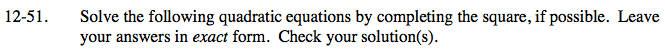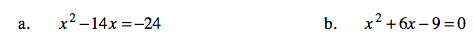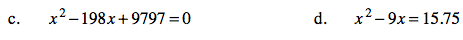### Home > AC > Chapter 12 > Lesson 12.2.2 > Problem12-51

12-51.x² − 14x + 49 = −24 + 49

Simplify. (x − 7)² = 25

Write two equations.

x − 7 = 5 x − 7 = −5

x = 12 or −2